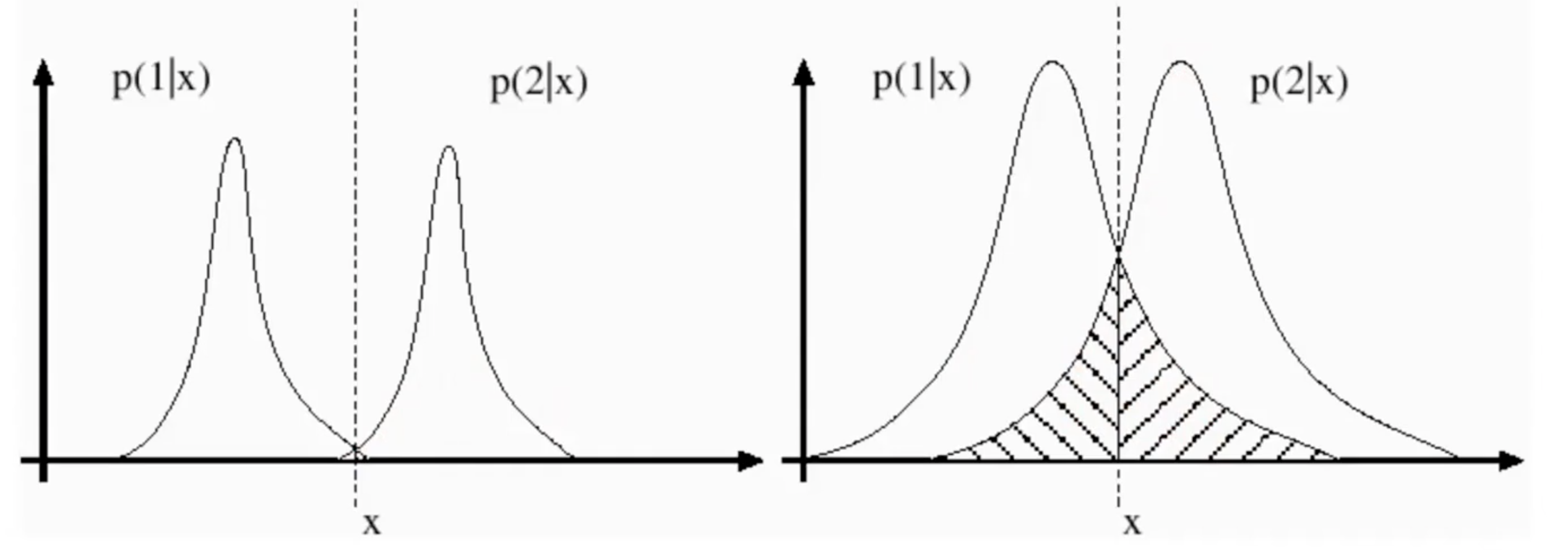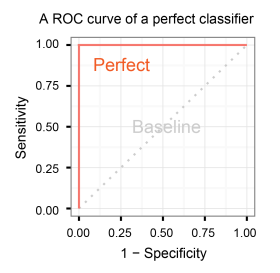## Data to Model §

### Random Sample Consensus (RANSAC) §

1. randomly choose minimal subset of data points necessary to fit model
2. points within some distance threshold of model are a consensus set, the size of the consensus set is the model’s support
3. repeat for N samples, model with biggest support is most robust fit

Choosing number of samples

1. let be the fraction of inliers
2. let be the number of points needed to define hypothesis (e.g. for a line)
3. suppose samples of points are chosen. then
1. the probability that all in a sample are correct is
4. the probability that all samples fail is , thus we choose a large enough to keep this below a targe failure rate

• general method
• easy to implement and calculate failure rate Disadvantages
• only handles a moderate percentage of outliers without cost blowing up
• many real problems have high rate of outliers (e.g. noise)

### Hough Transform §

• For each token, vote for all models to which the token could belong
• Return model with most votes

e.g. for each point, vote for all lines that could pass through it; true lines will pass through many points and thus receive many votes

Turning image space into parameter space. Rearranging into where and are the variables instead of and .

We can alternative transform it using Book’s Convention: . Then,

• Can handle high percentage of outliers: each point votes separately
• Can detect multiple instances of a model in a single pass Disadvantages:
• Complexity of search time increases exponentially with the number of model parameters
• Can be tricky to pick a good bin size

## Classification §

Classifier is a procedure that accepts as input a set of features and outputs a prediction for the class label.

### Standard Bag-of-Words pipeline §

1. Dictionary Learning: learn visual words using clustering
2. Encode: build Bags-of-words vectors for each image
3. Classify: train and test data using BOW (KNN, naive Bayes, SVM)

### Bayes Rule §

See: Bayes’ Theorem

Decision boundary, the location where one class becomes more probable than the other (e.g the point where the probability classes are equal).

The Bayes’ risk is the shaded region where one class’s probability is still non-zero beyond its decision boundary.### ROC Curve §

Trade-off between true positive rate and false positive rate. A random classifier will always have 1:1 true positive and false positive rate### Parametric vs Non-parametric §

• Parametric classifiers rely on a model
• fast, compact
• flexibility and accuracy depend on model assumptions
• Non-parametric classifiers are data driven (rely on comparing to training examples directly)
• slow
• highly flexible decision boundaries

### Spatial Pyramid §

Have multiple scales of the input image to compute histograms across. Train a classifier for each scale along with a combined weight to combine each classifier.

### VLAD (Vector of Locally Aggregated Descriptors) §

Instead of incrementing the histogram bin by a single count, we increment it by the residual vector (diff between cluster center and feature vector)

Dimensionality is where is number of codewords and is the dimensionality of the local descriptor (128 for SIFT)

## Decision Tree §

See notes on decision trees

### Classifier Boosting §

• Train an ensemble of classifiers sequentially
• Bias subsequent classifiers to correctly predict training examples that previous classifiers got wrong

## CNNs §

See notes on CNNs